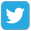Prepare forOlympiad Redeem Vouchers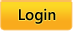Common Core:  MATH |  LANGUAGE ARTS |  SCIENCE
 Grades: Kindergarten |  First |  Second |  Third |  Fourth |  Fifth

# Common Core

Skills available for Common Core fifth-grade math standards

Click on the name of a skill to practice that skill.

### Operations and Algebraic Thinking.

Write and interpret numerical expressions.
• CC.5.OA.1 Use parentheses, brackets, or braces in numerical expressions, and evaluate expressions with these symbols.
• CC.5.OA.2 Write simple expressions that record calculations with numbers, and interpret numerical expressions without evaluating them. For example, express the calculation “add 8 and 7, then multiply by 2” as 2 × (8 + 7). Recognize that 3 × (18932 + 921) is three times as large as 18932 + 921, without having to calculate the indicated sum or product.

Analyze patterns and relationships. Generate two numerical patterns using two given rules.
• CC.5.OA.3 Identify apparent relationships between corresponding terms. Form ordered pairs consisting of corresponding terms from the two patterns, and graph the ordered pairs on a coordinate plane. For example, given the rule “Add 3” and the starting number 0, and given the rule “Add 6” and the starting number 0, generate terms in the resulting sequences, and observe that the terms in one sequence are twice the corresponding terms in the other sequence. Explain informally why this is so.

### Number and Operations in Base Ten

Understand the place value system.
• CC.5.NBT.1 Recognize that in a multi-digit number, a digit in one place represents 10 times as much as it represents in the place to its right and 1/10 of what it represents in the place to its left.
• CC.5.NBT.2 Explain patterns in the number of zeros of the product when multiplying a number by powers of 10, and explain patterns in the placement of the decimal point when a decimal is multiplied or divided by a power of 10. Use whole number exponents to denote powers of 10.

• CC.5.NBT.3 Read, write, and compare decimals to thousandths.

• CC.5.NBT.3a Read and write decimals to thousandths using base-ten numerals, number names, and expanded form, e.g., 347.392 = 3 × 100 + 4 × 10 + 7 × 1 + 3 × (1/10) + 9 × (1/100) + 2 × (1/1000).
• CC.5.NBT.3b Compare two decimals to thousandths based on meanings of the digits in each place, using >, =, and < symbols to record the results of comparisons.

Understand the place value system.
• CC.5.NBT.4 Use place value understanding to round decimals to any place.

Perform operations with multi-digit whole numbers and with decimals to hundredths.
• CC.5.NBT.5 Fluently multiply multi-digit whole numbers using the standard algorithm.

Perform operations with multi-digit whole numbers and with decimals to hundredths.
• CC.5.NBT.6 Find whole-number quotients of whole numbers with up to four-digit dividends and two-digit divisors, using strategies based on place value, the properties of operations, and/or the relationship between multiplication and division. Illustrate and explain the calculation by using equations, rectangular arrays, and/or area models.

• CC.5.NBT.7 Add, subtract, multiply, and divide decimals to hundredths, using concrete models or drawings and strategies based on place value, properties of operations, and/or the relationship between addition and subtraction; relate the strategy to a written method and explain the reasoning used.

### Number and Operations—Fractions

Use equivalent fractions as a strategy to add and subtract fractions.
• CC.5.NF.1 Add and subtract fractions with unlike denominators (including mixed numbers) by replacing given fractions with equivalent fractions in such a way as to produce an equivalent sum or difference of fractions with like denominators. For example, 2/3 + 5/4 = 8/12 + 15/12 = 23/12. (In general, a/b + c/d = (ad + bc)/bd.)

• CC.5.NF.2 Solve word problems involving addition and subtraction of fractions referring to the same whole, including cases of unlike denominators, e.g., by using visual fraction models or equations to represent the problem. Use benchmark fractions and number sense of fractions to estimate mentally and assess the reasonableness of answers. For example, recognize an incorrect result 2/5 + 1/2 = 3/7 by observing that 3/7 < 1/2.

Apply and extend previous understandings of multiplication and division to multiply and divide fractions.
• CC.5.NF.3 Interpret a fraction as division of the numerator by the denominator (a/b = a ÷ b). Solve word problems involving division of whole numbers leading to answers in the form of fractions or mixed numbers, e.g., by using visual fraction models or equations to represent the problem. For example, interpret 3/4 as the result of dividing 3 by 4, noting that 3/4 multiplied by 4 equals 3 and that when 3 wholes are shared equally among 4 people each person has a share of size 3/4. If 9 people want to share a 50-pound sack of rice equally by weight, how many pounds of rice should each person get? Between what two whole numbers does your answer lie?
• CC.5.NF.4 Apply and extend previous understandings of multiplication to multiply a fraction or whole number by a fraction.
• CC.4.NF.3a Understand addition and subtraction of fractions as joining and separating parts referring to the same whole.
• CC.5.NF.4a Interpret the product (a/b) × q as a parts of a partition of q into b equal parts; equivalently, as the result of a sequence of operations a × q ÷ b. For example, use a visual fraction model to show (2/3) × 4 = 8/3, and create a story context for this equation. Do the same with (2/3) × (4/5) = 8/15. (In general, (a/b) × (c/d) = ac/bd.)
• CC.5.NF.4b Find the area of a rectangle with fractional side lengths by tiling it with unit squares of the appropriate unit fraction side lengths, and show that the area is the same as would be found by multiplying the side lengths. Multiply fractional side lengths to find areas of rectangles, and represent fraction products as rectangular areas.

Apply and extend previous understandings of multiplication and division to multiply and divide fractions.
• CC.5.NF.5 Interpret multiplication as scaling (resizing) by: -- a. Comparing the size of a product to the size of one factor on the basis of the size of the other factor, without performing the indicated multiplication. -- b. Explaining why multiplying a given number by a fraction greater than 1 results in a product greater than the given number (recognizing multiplication by whole numbers greater than 1 as a familiar case); explaining why multiplying a given number by a fraction less than 1 results in a product smaller than the given number; and relating the principle of fraction equivalence a/b = (n×a) / (n×b) to the effect of multiplying a/b by 1.
• CC.5.NF.6 Solve real world problems involving multiplication of fractions and mixed numbers, e.g., by using visual fraction models or equations to represent the problem.
• CC.5.NF.7 Apply and extend previous understandings of division to divide unit fractions by whole numbers and whole numbers by unit fractions. (Students able to multiply fractions in general can develop strategies to divide fractions in general, by reasoning about the relationship between multiplication and division. But division of a fraction by a fraction is not a requirement at this grade.)
• CC.5.NF.7a Interpret division of a unit fraction by a non-zero whole number, and compute such quotients. For example, create a story context for (1/3) ÷ 4 and use a visual fraction model to show the quotient. Use the relationship between multiplication and division to explain that (1/3) ÷ 4 = 1/12 because (1/12) × 4 = 1/3.

• CC.5.NF.7b Interpret division of a whole number by a unit fraction, and compute such quotients. For example, create a story context for 4 ÷ (1/5) and use a visual fraction model to show the quotient. Use the relationship between multiplication and division to explain that 4 ÷ (1/5) = 20 because 20 × (1/5) = 4.
• CC.5.NF.7c Solve real-world problems involving division of unit fractions by non-zero whole numbers and division of whole numbers by unit fractions, e.g., by using visual fraction models and equations to represent the problem. For example, how much chocolate will each person get if 3 people share 1/2 lb of chocolate equally? How many 1/3-cup servings are in 2 cups of raisins?

### Measurement and Data

Convert like measurement units within a given measurement system.
• CC.5.MD.1 Convert among different-sized standard measurement units within a given measurement system (e.g., convert 5 cm to 0.05 m), and use these conversions in solving multi-step real world problems.

Represent and interpret data. Make a line plot to display a data set of measurements in fractions of a unit (1/2, 1/4, 1/8)
• CC.5.MD.2 Use operations on fractions for this grade to solve problems involving information presented in line plots. For example, given different measurements of liquid in identical beakers, find the amount of liquid each beaker would contain if the total amount in all the beakers were redistributed equally.

Geometric measurement: understand concepts of volume and relate volume to multiplication and to addition.
• CC.5.MD.3 Recognize volume as an attribute of solid figures and understand concepts of volume measurement. -- a. A cube with side length 1 unit, called a “unit cube,” is said to have “one cubic unit” of volume, and can be used to measure volume. -- b. A solid figure which can be packed without gaps or overlaps using n unit cubes is said to have a volume of n cubic units.

• CC.5.MD.4 Measure volumes by counting unit cubes, using cubic cm, cubic in, cubic ft, and improvised units.

• CC.5.MD.5 Relate volume to the operations of multiplication and addition and solve real world and mathematical problems involving volume.
• CC.5.MD.5a Find the volume of a right rectangular prism with whole-number side lengths by packing it with unit cubes, and show that the volume is the same as would be found by multiplying the edge lengths, equivalently by multiplying the height by the area of the base. Represent three-fold whole-number products as volumes, e.g., to represent the associative property of multiplication.
• CC.5.MD.5b Apply the formulas V =(l)(w)(h) and V = (b)(h) for rectangular prisms to find volumes of right rectangular prisms with whole-number edge lengths in the context of solving real world and mathematical problems.
• CC.5.MD.5c Recognize volume as additive. Find volumes of solid figures composed of two non-overlapping right rectangular prisms by adding the volumes of the non-overlapping parts, applying this technique to solve real world problems.

### Geometry

Graph points on the coordinate plane to solve real-world and mathematical problems.
• CC.5.G.1 Use a pair of perpendicular number lines, called axes, to define a coordinate system, with the intersection of the lines (the origin) arranged to coincide with the 0 on each line and a given point in the plane located by using an ordered pair of numbers, called its coordinates. Understand that the first number indicates how far to travel from the origin in the direction of one axis, and the second number indicates how far to travel in the direction of the second axis, with the convention that the names of the two axes and the coordinates correspond (e.g., x-axis and x-coordinate, y-axis and y-coordinate).
• CC.5.G.2 Represent real world and mathematical problems by graphing points in the first quadrant of the coordinate plane, and interpret coordinate values of points in the context of the situation.

Classify two-dimensional figures into categories based on their properties.
• CC.5.G.3 Understand that attributes belonging to a category of two-dimensional figures also belong to all subcategories of that category. For example, all rectangles have four right angles and squares are rectangles, so all squares have four right angles.

Classify two-dimensional figures into categories based on their properties.
• CC.5.G.4 Classify two-dimensional figures in a hierarchy based on properties.

 Connect and Follow About Intelliseeds Learning My Account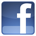Facebook Benefits Membership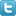Twitter Plans and Pricing Skills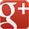Google+ FAQ Wall of Fame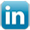LinkedIn Newsletter Student Reports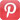Pinterest Careers Rewards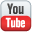Youtube Contact Us What is IntelliAbility?Our Partners Terms & Conditions Tell a FriendNon Profit Partners Testimonials User Guide PTA Fundraising Offers Common Core Math Schools Common Core ELA Virginia SOL Content Team
 Welcome to Intelliseeds Learning Smart learning for your child Intelliseeds learning provides practice in several subjects including Math, Reading Comprehension, Language Arts, Logical Reasoning, Mental Ability, Analytical Ability and Critical Thinking, which are essential to the success of every student. Skills are organized by grades, topics, practice tests and time bound tests. The Math section covers skills such as counting, number operations, algebra, story problems and many more. The IntelliAbility section focuses on thinking skills, patterns, sequences, problem analysis, analogies and over 50 other topics to help develop your child's brain. Click here to learn more about IntelliAbility. The Language Arts section includes reading comprehension, nouns, verbs, adverbs, adjectives, predicates, vowel sounds and many more grammar skills.Privacy | © 2022 Intelliseeds Learning™